#### You may also like### Summing Consecutive Numbers

15 = 7 + 8 and 10 = 1 + 2 + 3 + 4. Can you say which numbers can be expressed as the sum of two or more consecutive integers?### Always the Same

Arrange the numbers 1 to 16 into a 4 by 4 array. Choose a number. Cross out the numbers on the same row and column. Repeat this process. Add up you four numbers. Why do they always add up to 34?### Fibs

The well known Fibonacci sequence is 1 ,1, 2, 3, 5, 8, 13, 21.... How many Fibonacci sequences can you find containing the number 196 as one of the terms?

# Beach Huts

##### Age 11 to 14Challenge Level

Thank you and well done to everyone who submitted solutions to this problem. Far too many of you sent in solutions to mention all of you, but there were lots and lots of good ideas.

Gavin, from Woodhaven School in Canada, described how the numbers in each hut are related:

The answer for the hut is the roof is the difference between the 2 blocks in the middle of the house. Then the two blocks in the middle added together makes the bottom number.

Ruby, Hannah and Emma, from Berkeley Primary School, used algebra to relate these numbers: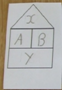They found the following relationships:
$\begin{eqnarray} B-A&=&X\\ B+A&=&Y \end{eqnarray}$

Jared, from Associated Hebrew Schools in Canada, was able to relate each hut to the subsequent hut:

$X$ is always the same as $A$ in the next hut. $Y$ is always the same as $B$ in the next hut.

Ruby, Hannah and Emma described this pictorially: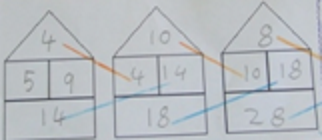Many of you were able to find the next few huts using these rules: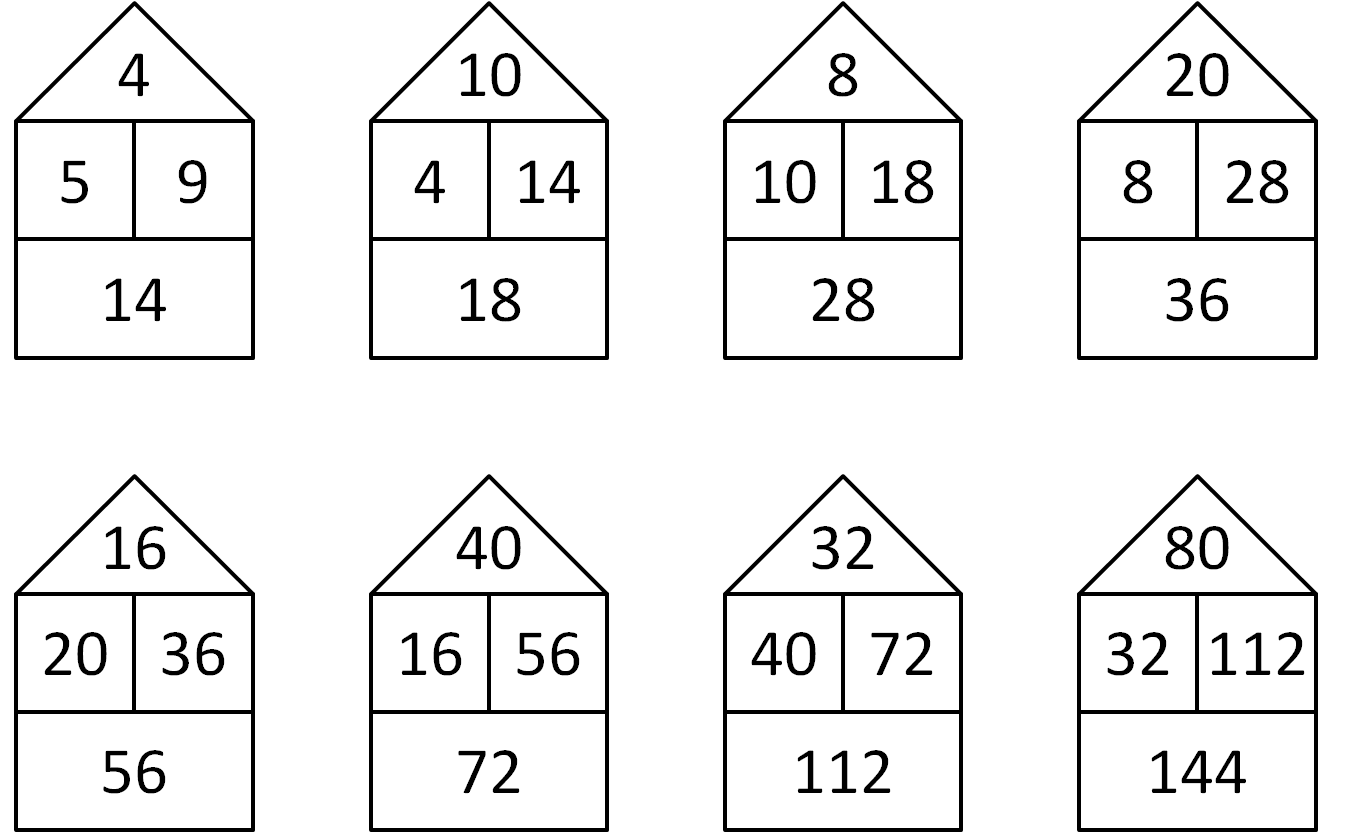Tom, Morgan and Libby, from Berkeley Primary School, had a different way of explaining the patterns in the beach huts.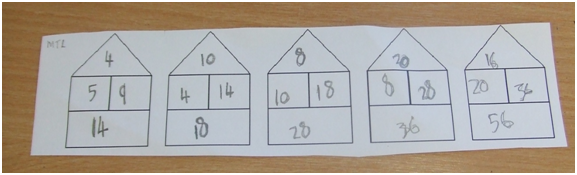The 5 swaps places with the 4, and the 5 then doubles.

As for the 9 and the 14, they swap too, and the nine doubles. Now you have the numbers in place for the next hut. Then you do the same for the next hut, just with different numbers.

Mackenzie, from Queen Elizabeth's Hospital School, noticed a pattern when you move two stacks to the right:

The odd term huts follow a pattern and the even term huts also follow a pattern. For example, if you knew the fifth term hut then you could easily find the seventh hut by doubling all of the numbers in the fifth. This also worked for the even term huts, e.g. tenth hut is double the eighth. Therefore in order to find the 100th term (even number) we needed to double the numbers in the second hut 50 times.

Patrick, from Hitchin Boy's School, was then able to express the value of the numbers in the 100th hut to the right algebraically:

You have to move to the right 100 huts. Since moving two to the right doubles the number, you need to multiply the numbers in the second hut by two 50 times, or by $2^{50}$.

Kelly and Beth, from Loughborough High School, used algebra to check that this doubling always takes place: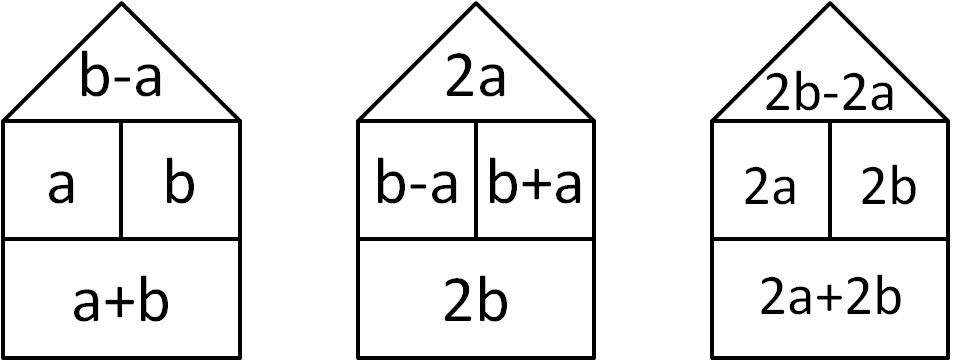The third beach hut is then always twice the third beach hut, so moving two huts to the right always doubles the number.

Imesh, from Roding Primary School, was able to describe how to go backwards from one hut to the previous one:

To find the beach hut before the one given, the left number in the middle floor is the same as the roof in the hut before it and the bottom floor in the hut before is equal to the number of the right number in the middle floor in the given hut. As you found the bottom and top numbers, to find the left middle floor number in the hut before you do the bottom number subtract the top number divided by two. Finally, to get the right number in the middle floor, you should subtract the left middle-floor number from the bottom number.

7Y, from Queen Elizabeth's Hospital School, were able to explain how to go back many huts to the left.

After this we considered going in the opposite direction (to the left) and quickly deduced that we just needed to reverse
the operation of  (doubling) and divide each number by two instead in order to move left by two huts.

Zach was able to use this to find many of the different terms. You can see his solution here, and the second part here .